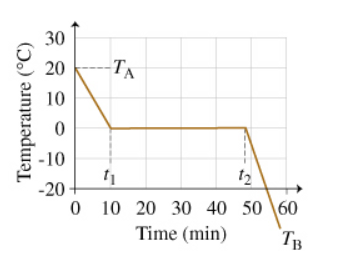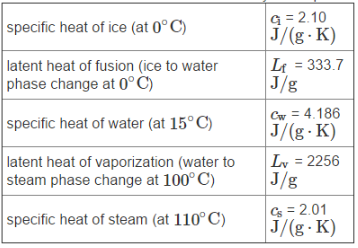# Problem: Refer to the temperature versus time graph (Figure 2) when answering the questions in Parts C through F A system consists of 250 g of water. The system, originally at TA 21.0 °C, is placed in a freezer, where energy is removed from it in the form of heat at a constant rate. The figure shows how the temperature of the system takes t1  = 10 min 600 s to drop to 0°C, after which the water freezes. Once the freezing is complete, the temperature of the resulting ice continues to drop, reaching temperature TB after an hour. The following specific heat and latent heat values for water may be helpful.How much energy must be transferred out of the system as heat Q to lower its temperature to 0°C?Cooling Power of P is 36.6 WAt what time t2 will the water be completely frozen so the temperature can begin to fall below °C?(seconds)

###### FREE Expert Solution

Heat:

$\overline{){\mathbf{Q}}{\mathbf{=}}{\mathbf{mC}}{\mathbf{∆}}{\mathbf{T}}}$

Heat of fusion:

$\overline{){\mathbf{Q}}{\mathbf{=}}{\mathbf{m}}{{\mathbf{L}}}_{{\mathbf{f}}}}$

Power:

$\overline{){\mathbf{P}}{\mathbf{=}}\frac{\mathbf{E}}{\mathbf{t}}}$

(a)

Heat lost while cooling water to 0 °C:

Cw = 4.186 J/(g•K) × (1000g/kg) = 4186 J/(kg•K)

m = 250 g (1kg/1000g) = 0.25kg

1 °C = 1 K

ΔT = (21 °C - 0 °C) = 21 °C (1K/1 °C) = 21 K

82% (98 ratings)###### Problem DetailsRefer to the temperature versus time graph (Figure 2) when answering the questions in Parts C through F A system consists of 250 g of water. The system, originally at TA 21.0 °C, is placed in a freezer, where energy is removed from it in the form of heat at a constant rate. The figure shows how the temperature of the system takes t1  = 10 min 600 s to drop to 0°C, after which the water freezes. Once the freezing is complete, the temperature of the resulting ice continues to drop, reaching temperature TB after an hour. The following specific heat and latent heat values for water may be helpful.How much energy must be transferred out of the system as heat Q to lower its temperature to 0°C?

Cooling Power of P is 36.6 W

At what time t2 will the water be completely frozen so the temperature can begin to fall below °C?

(seconds)